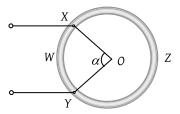NEET Physics Current Electricity Questions Solved

A wire of resistor R is bent into a circular ring of radius r. Equivalent resistance between two points X and Y on its circumference, when angle XOY is α, can be given by(1) $\frac{R\alpha }{4{\pi }^{2}}\left(2\pi -\alpha \right)$

(2) $\frac{R}{2\pi }\left(2\pi -\alpha \right)$

(3) R (2πα)

(4) $\frac{4\pi }{R\alpha }\left(2\pi -\alpha \right)$

Explanation is a part of a Paid Course. To view Explanation Please buy the course.

Difficulty Level: